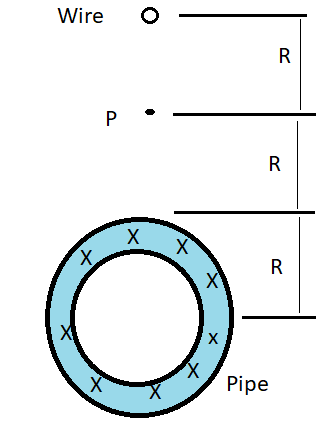# In the figure below, a long circular pipe with outside radius R = 2.17 cm carries a (uniformly...

## Question:

In the figure below, a long circular pipe with outside radius R = 2.17 cm carries a (uniformly distributed) current i = 10.4 mA into the page. A wire runs parallel to the pipe at a distance of 3.00R from center to center. Find the (a) magnitude and (b) direction (into or out of the page) of the current in the wire such that the ratio of the magnitude of the net magnetic field at point P to the magnitude of the net magnetic field at the center of the pipe is 4.82, but it has the opposite direction.## Net Magnetic Field Near Two Current-Carrying Wires

The net magnetic field at a point near two current-carrying wires is calculated by adding the magnetic fields at that point due to each individual current-carrying wires if they are at the same direction or subtracting the magnetic fields at that point due to each individual magnetic field if they are at the different directions.

The magnitude of the magnetic field at a point near a current-carrying wire is given by:

{eq}B\ =\ \dfrac{\mu_0I}{2\pi r}\\ {/eq}

The direction of the magnetic field is determined by the right-hand rule.

We are given:

• {eq}R\ =\ 2.17\ cm {/eq} = outside radius of the circular pipe.
• {eq}I\ =\ 10.4\ A {/eq} = current through the pipe.
• {eq}d\ =\...

Become a Study.com member to unlock this answer! Create your account